# 4.1 Derivation of the two-dimensional Helmholtz equation model

Figure 4.1 depicts a cross-section of the volume between a PCB ground plane and a metallic cover. This volume contains the metallic layout structures on the PCB, the active and passive components, metallic cooling structures, thin sheets of PCB dielectric material, and for the most part air. In case of a PCB stack the metallic cover plane is given by the ground plane of the parallel PCB.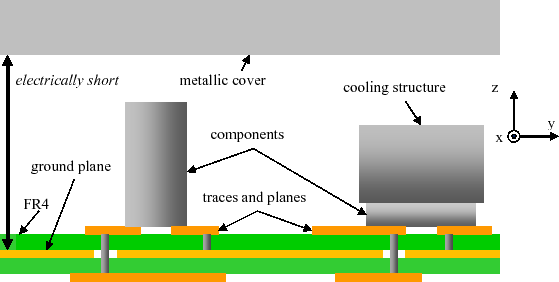All metallic PCB layout structures, the components on the PCB, and metallic cooling devices will be introduced into the field formulation by excitation ports in a second step and, thus, these parts are neglected for the derivation of the cavity field equation. Therefore, the field is derived for a volume that contains only isolating dielectric layers. The first and the third Maxwell equations for harmonic signals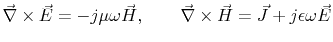(4.1)

are combined to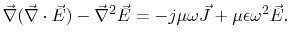(4.2)

Where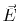is the electrical field strength,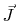is the current density,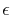is the permittivity of the layer,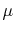is the permeability of the layer and, with the frequency f.
The dielectric layers of such applications (air, FR4,...) are usually homogenous regarding their macroscopic electrical parameters. Therefore, there are no charges (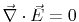) inside one layer and the first term of (4.2) vanishes, leading to the three-dimensional, vectorial Helmholtz equation(4.3)

Equation (4.3) is general for homogenous materials without volume charges. It can be reduced to a scalar two-dimensional Helmholtz equation by the following conditions:

 Perfect conducting cover and ground plane: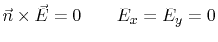(4.4)

 Electrically small cover to ground plane distance: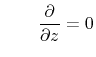(4.5)

Condition (4.4) is reasonable for emission simulation of enclosures, because the main loss mechanism is the radiation loss from the enclosure slots, which dominates compared to the conduction loss of the metallic planes . However, conduction and dielectric losses will be considered in the cavity model, without noticeable violation of the condition.
Condition (4.5) limits the frequency range for the model. Table 4.1 list the frequency limits for some cover to ground plane distances based on the often used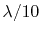lumped element criterion and the more tolerable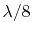condition for short antennas. The table demonstrates that the whole CISPR25 frequency range of 2.5GHz is covered up to a plane separation of 15mm and nearly covered up to 20mm. The maximum frequency limit is beyond 1 GHz even at a plane separation distance of 30mm.

Table 4.1: Frequency limits of the cavity model for several plane separation distances.
 Plane separation (mm) 3 5 7 10 12 15 20 30limit (GHz) 10 6 4.3 3 2.5 2 1.5 1limit (GHz) 12.5 7.5 5.4 3.8 3.1 2.5 1.9 1.3

The two-dimensional, scalar, and lossless Helmholtz equation for the cavity field inside the enclosure is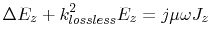with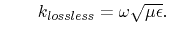(4.6)

A formulation for the voltage U on the planes is obtained by multiplying (4.6) with the plane separation distance h: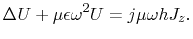(4.7)

Equation (4.6) is expressed as a two-dimension transmission line equation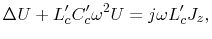(4.8)

with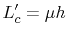and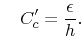(4.9)

According to transmission line theory, the introduction of conduction and dielectric losses into (4.8) yields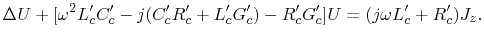(4.10)

The surface resistance which considers conduction losses is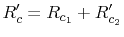where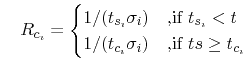with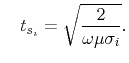(4.11)

Where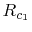and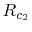are the surface resistances of the lower and upper plane respectively. With i=1 and i=2 for the two planes,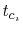is the plane thickness,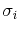is the conductivity of the plane metal, and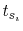is the skin depth of the plane. The dielectric losses of the isolation layer are considered in (4.10) by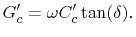(4.12)

Where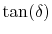is the loss tangent of the material. To meet the requirement (4.4), the term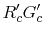of (4.10) must be small compared to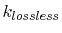. On the right hand side term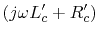,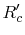must be small compared to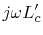. This is in fact the case for the indented application of the method, because of the highly conducting metallic planes and the significant portion of air in the volume. Therefore, these terms can be neglected. With this simplification (4.9) and (4.12), the equation for the voltage distribution inside the cavity, becomes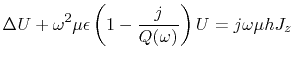with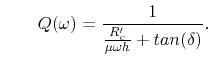(4.13)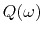is the total quality factor which considers the conduction and the dielectric losses.
Finally, the Helmholtz equation for the electric field strength, which considers conduction and dielectric losses is(4.14)

Subsections

C. Poschalko: The Simulation of Emission from Printed Circuit Boards under a Metallic Cover